ZHCSG66 March   2017

PRODUCTION DATA.

1. 特性
2. 应用
3. 说明
4. 修订历史记录
5. Pin Configuration and Functions
6. Specifications
7. Parameter Measurement Information
8. Detailed Description
1. 8.1 Overview
2. 8.2 Functional Block Diagram
3. 8.3 Feature Description
4. 8.4 Device Functional Modes
1. 8.4.1 ACQ State
2. 8.4.2 CNV State
3. 8.4.3 OFFCAL State
9. Application and Implementation
1. 9.1 Application Information
2. 9.2 Typical Applications
1. 9.2.1 Single-Supply Data Acquisition With the ADS7056
2. 9.2.2 High Bandwidth (1 MHz) Data Acquisition With the ADS7056
3. 9.2.3 14-Bit, 10-kSPS DAQ Circuit Optimized for DC Sensor Measurements
10. 10Power Supply Recommendations
11. 11Layout
12. 12器件和文档支持
1. 12.1 文档支持
2. 12.2 接收文档更新通知
3. 12.3 社区资源
4. 12.4 商标
5. 12.5 静电放电警告
6. 12.6 Glossary
13. 13机械、封装和可订购信息

• RUG|8

## 6 Specifications

### 6.1 Absolute Maximum Ratings(1)

MIN MAX UNIT
AVDD to GND –0.3 3.9 V
DVDD to GND –0.3 3.9 V
AINP to GND –0.3 AVDD + 0.3 V
AINM to GND –0.3 0.3 V
Input current to any pin except supply pins –10 10 mA
Digital input voltage to GND –0.3 DVDD + 0.3 V
Storage temperature, Tstg –60 150 °C
Stresses beyond those listed under Absolute Maximum Ratings may cause permanent damage to the device. These are stress ratings only, which do not imply functional operation of the device at these or any other conditions beyond those indicated under Recommended Operating Conditions. Exposure to absolute-maximum-rated conditions for extended periods may affect device reliability.

### 6.2 ESD Ratings

VALUE UNIT
V(ESD) Electrostatic discharge Human body model (HBM), per ANSI/ESDA/JEDEC JS-001(1) ±2000 V
Charged device model (CDM), per JEDEC specification JESD22-C101(2) ±1000
JEDEC document JEP155 states that 500-V HBM allows safe manufacturing with a standard ESD control process.
JEDEC document JEP157 states that 250-V CDM allows safe manufacturing with a standard ESD control process.

### 6.3 Recommended Operating Conditions

over operating free-air temperature range (unless otherwise noted)
MIN NOM MAX UNIT
AVDD Analog supply voltage range 2.35 3 3.6 V
DVDD Digital supply voltage range 1.65 1.8 3.6 V
TA Operating free-air temperature –40 25 125 °C

### 6.4 Thermal Information

RUG (X2QFN)
8 PINS
RθJA Junction-to-ambient thermal resistance 177.5 °C/W
RθJC(top) Junction-to-case (top) thermal resistance 51.5 °C/W
RθJB Junction-to-board thermal resistance 76.7 °C/W
ψJT Junction-to-top characterization parameter 1 °C/W
ψJB Junction-to-board characterization parameter 76.7 °C/W
RθJC(bot) Junction-to-case (bottom) thermal resistance N/A °C/W

### 6.5 Electrical Characteristics

at AVDD = 3.3 V, DVDD = 1.65 V to 3.6 V, fSAMPLE = 2.5 MSPS, and VAINM = 0 V (unless otherwise noted); minimum and maximum values for TA = –40°C to +125°C; typical values at TA = 25°C
PARAMETER TEST CONDITIONS MIN TYP MAX UNIT
Full-scale input voltage span(1) 0 AVDD V
Absolute input voltage range AINP to GND –0.1 AVDD + 0.1 V
AINM to GND –0.1 0.1
CS Sampling capacitance 16 pF
SYSTEM PERFORMANCE
Resolution 14 Bits
NMC No missing codes 14 Bits
INL(8) Integral nonlinearity –3 ±2 3 LSB(2)
DNL Differential nonlinearity –0.99 ±0.5 1 LSB
EO (8) Offset error After calibration(7) –6 ±2.5 6 LSB
dVOS/dT Offset error drift with temperature 1.75 ppm/°C
EG(8) Gain error –0.1 ±0.01 0.1 %FS
Gain error drift with temperature 0.5 ppm/°C
SAMPLING DYNAMICS
tCONV Conversion time 18 × tSCLK ns
tACQ Acquisition time 95 ns
fSAMPLE Maximum throughput rate 60-MHz SCLK, AVDD = 2.35 V to 3.6 V 2.5 MHz
Aperture delay 3 ns
Aperture jitter, RMS 12 ps
DYNAMIC CHARACTERISTICS
SNR Signal-to-noise ratio(4) AVDD = 3.3 V 72 74.9 dB
AVDD = 2.5 V 73.7
THD Total harmonic distortion(4)(3) fIN = 2 kHz 85 dB
fIN = 250 kHz 84.8
fIN = 1000 kHz 84.5
SINAD Signal-to-noise and distortion(4) fIN = 2 kHz 71.75 74.5 dB
fIN = 250 kHz 73.7
fIN = 1000 kHz 73.7
SFDR Spurious-free dynamic range(4) fIN = 2 kHz 89.8 dB
fIN = 250 kHz 88
fIN = 1000 kHz 87.5
BW(fp) Full-power bandwidth At –3 dB 200 MHz
DIGITAL INPUT/OUTPUT (CMOS Logic Family)
VIH High-level input voltage(5) 0.65 DVDD DVDD + 0.3 V
VIL Low-level input voltage(5) –0.3 0.35 DVDD V
VOH High-level output voltage(5) At Isource = 500 µA 0.8 DVDD DVDD V
At Isource = 2 mA DVDD – 0.45 DVDD
VOL Low-level output voltage(5) At Isink = 500 µA 0 0.2 DVDD V
At Isink = 2 mA 0 0.45
POWER-SUPPLY REQUIREMENTS
AVDD Analog supply voltage 2.35 3 3.6 V
DVDD Digital I/O supply voltage 1.65 3 3.6 V
IAVDD Analog supply current AVDD = 3.3 V, fSAMPLE = 2.5 MSPS 1050 1250 µA
AVDD = 3.3 V, fSAMPLE = 100 kSPS 48 50
AVDD = 3.3 V, fSAMPLE = 10 kSPS 5
AVDD = 2.5 V, fSAMPLE = 2.5 MSPS 750
Static current with CS and SCLK high 0.02
IDVDD Digital supply current DVDD = 1.8 V, CSDO = 20 pF,
output code = 2AAAh(6)
630 µA
DVDD = 1.8 V, static current with CS and SCLK high 0.01
Ideal input span; does not include gain or offset error.
LSB means least significant bit.
Calculated on the first nine harmonics of the input frequency.
All specifications expressed in decibels (dB) refer to the full-scale input (FSR) and are tested with an input signal 0.5 dB below full-scale, unless otherwise noted.
Digital voltage levels comply with the JESD8-7A standard for DVDD from 1.65 V to 1.95 V; see the Parameter Measurement Information section for details.
See the Estimating Digital Power Consumption section for details.
See the OFFCAL State section for details.
See Figure 32, Figure 33, and Figure 34 for statistical distribution data for INL, offset error, and gain error.

### 6.6 Timing Requirements

all specifications are at AVDD = 2.35 V to 3.6 V, DVDD = 1.65 V to 3.6 V, and CLOAD-SDO = 20 pF (unless otherwise noted); minimum and maximum values for TA = –40°C to +125°C; typical values at TA = 25°C
MIN TYP MAX UNIT
tCLK Time period of SCLK 16.66 ns
tsu_CSCK Setup time: CS falling edge to SCLK falling edge 7 ns
tht_CKCS Hold time: SCLK rising edge to CS rising edge 8 ns
tph_CK SCLK high time 0.45 0.55 tSCLK
tpl_CK SCLK low time 0.45 0.55 tSCLK
tph_CS CS high time 15 ns

### 6.7 Switching Characteristics

all specifications are at AVDD = 2.35 V to 3.6 V, DVDD = 1.65 V to 3.6 V, and CLOAD-SDO = 20 pF (unless otherwise noted); minimum and maximum values for TA = –40°C to +125°C; typical values at TA = 25°C
PARAMETER TEST CONDITIONS MIN TYP MAX UNIT
tCYCLE(1) Cycle time 400 ns
tCONV Conversion time 18 × tSCLK ns
tden_CSDO Delay time: CS falling edge to data enable 6.5 ns
td_CKDO Delay time: SCLK rising edge to (next) data valid on SDO 10 ns
tht_CKDO SCLK rising edge to current data invalid 2.5
tdz_CSDO Delay time: CS rising edge to SDO going to tri-state 5.5 ns
tCYCLE = 1 / fSAMPLE.Figure 1. Serial Transfer FrameFigure 2. Timing Specifications

### 6.8 Typical Characteristics

at TA = 25°C, AVDD = 3.3 V, DVDD = 1.8 V, fIN = 2 kHz, and fSample = 2.5 MSPS (unless otherwise noted)SNR = 75.2 dB, THD = –90.25 dB, ENOB = 12.18 bits
Figure 3. Typical FFTSNR = 74.2 dB, THD = –90.25 dB, fIN = 500 kHz
Figure 5. Typical FFT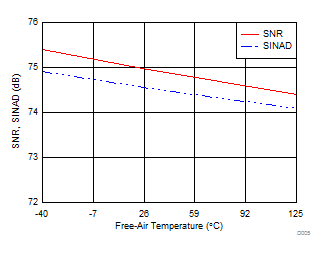Figure 7. SNR and SINAD vs Temperature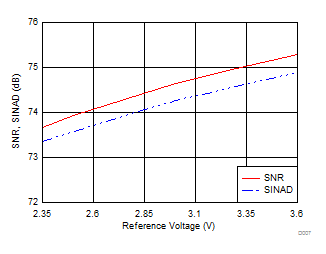Figure 9. SNR and SINAD vs Reference Voltage (AVDD)Figure 11. SFDR vs TemperatureFigure 13. SFDR vs Input Frequency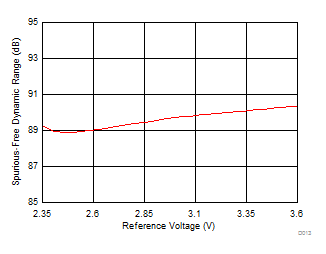Figure 15. SFDR vs Reference Voltage (AVDD)Figure 17. Offset vs TemperatureFigure 19. Gain Error vs Temperature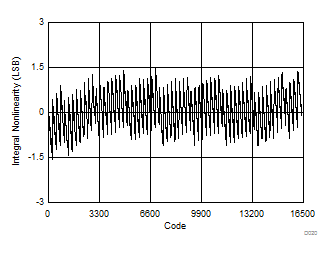Figure 21. Typical INLAVDD = 2.35 V
Figure 23. Typical INL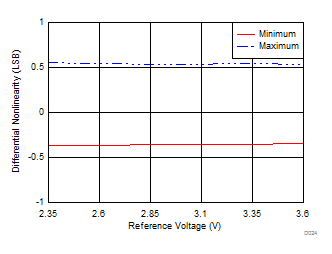Figure 25. DNL vs Reference VoltageFigure 27. INL vs Reference VoltageFigure 29. AVDD Current vs ThroughputCS = DVDD
Figure 31. Static AVDD Current vs Temperature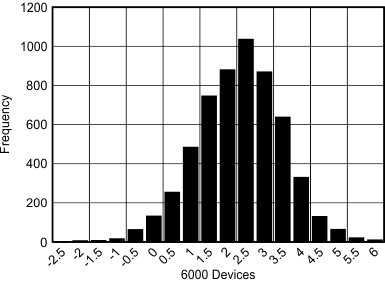Figure 33. Typical Offset Error DistributionSNR = 74.3 dB, THD = –87.9 dB, fIN = 250 kHz
Figure 4. Typical FFTSNR = 73.9 dB, THD = –87.1 dB, fIN = 1000 kHz
Figure 6. Typical FFT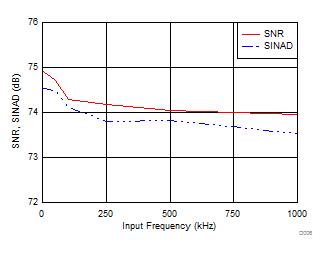Figure 8. SNR and SINAD vs Input FrequencyFigure 10. THD vs TemperatureFigure 12. THD vs Input FrequencyFigure 14. THD vs Reference Voltage (AVDD)Standard deviation of codes = 0.94 LSB, VIN = AVDD / 2
Figure 16. DC Input HistogramFigure 18. Offset vs Reference Voltage (AVDD)Figure 20. Typical DNLAVDD = 2.35 V
Figure 22. Typical DNLFigure 24. DNL vs Temperature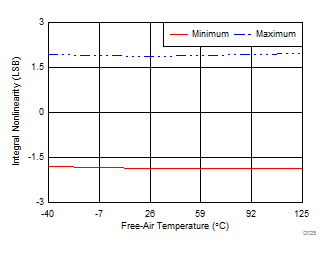Figure 26. INL vs Temperature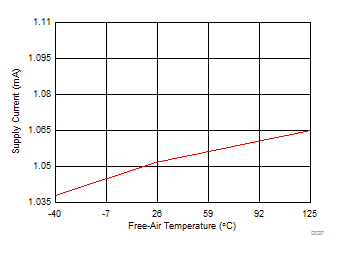Figure 28. AVDD Current vs Temperature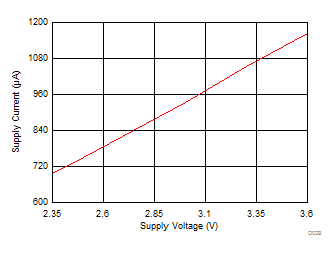Figure 30. AVDD Current vs AVDD Voltage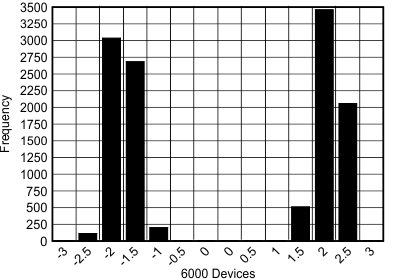Figure 32. Typical INL DistributionFigure 34. Typical Gain Error Distribution